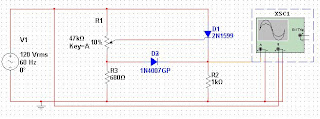Single Phase half wave thyristor circuit [simulation].

In some of my previous posts, we have seen the simulations of half wave and full wave rectifiers using simple diodes. Now in this post, I would like to move on to power electronics domain with the first post being “Single Phase half wave rectifier using thyristor”.

In a thyristor or a so called SCR (Silicon controlled rectifier) circuit, the thyristor is turned on after a delay angle alpha (α) using a firing circuit. α is also called firing angle.

So, in the circuit shown below, the SCR starts conducting only after a delay angle of α, which is controlled by the potentiometer across the Anode and Gate terminals of the SCR. When the SCR starts conduction, the voltage appears across the load resistor of 1kΩ as shown in graph-2, otherwise during off condition of SCR, the voltage appears only across the SCR as shown in graph-3.Circuit diagram/schematic of single phase half wave thyristor circuit (R-Load)

Note that these simulations were performed in the NI MultiSim software.Graph-1: Source Voltage
Graph-1 here depicts the source voltage. It can be easily inferred from the graph that the source voltage is sinusoidal.Graph-2: Voltage across the load

You can see the in the above graph that the voltage appears across the load only after a certain delay angle (firing angle). Conduction occurs only in positive half cycles, since during negative half cycles, the SCR is switched off.

Part of the sinusoidal waveform which do not appear across the load resistor appears across the thyristor as shown in the graph-3. These waveforms appear when the thyristor is on OFF modeGraph-3: Voltage across the thyristor

1.The Thyristors design is perfect and clear.

2.Really Nice Content on single phase Thyristors...Please suggest a article which provides more information on comparison between Reverse conducting & Light triggered Thyristors.....

3.Circuit is exact and output is clear.How to change firing angle in this circuit?

4.Circuit is exact and output is clear.How to change firing angle in this circuit?

If you liked this blog then Join me at: